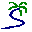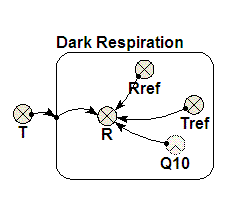# Model entry

Model of plant dark respiration as an exponential function of temperature parameterised by Q10 - Model catalogue - Simulistics.com

 Search Simulistics Model catalogue Listed by keyword Listed by ID Listed by title Listed by date added

# Model of plant dark respiration as an exponential function of temperature parameterised by Q10

Model : respirationQ10
Simile version : 3.1+
Keywords : Ecophysiology ; Carbon dynamics ;

## Description

Respiration here means the production of carbon dioxide as a by-product of the process by which energy in carbohydrate is released (in the form of ATP) in living things.

The basic ecophysiological respiration model is an exponential function of temperature (which has a physical basis in the Arrhenius equation).

Respiration rate, R = Ro * exp(k*t)
where:

• R is the respiration rate
• Ro is the respiration rate at zero Celsius
• t is the temperature (Celsius)
• k is a empirical constant
This is the basic ecophysiological dark plant respiration model and is also often used for soil respiration. Dark respiration is so named to differentiate it from light respiration (photorespiration) which only occurs in plants in the light and is part of the photosynthetic carbon reduction cycle. Dark respiration occurs in light and dark conditions in all cells.

## Files

### Model file

Click on the icon to download the model file. (You will need Simile to examine and run the model. A free evaluation version is available from the products page.)

Some browsers may attempt to display the model file, rather than open it in Simile; in this case, use the browser back button to return to this page, and use the context menu (invoked by right-clicking on the link) to save the target file to disk.respirationQ10.sml

## Diagram## Equations

``` Respiration rate,	R = Rref*Q10^((T-Tref)/10)
Where:
Rref is respiration rate at reference temperature Tref
Tref is the temperature where respiration rate equals Rref
Q10  is the coefficient by which R increases for every 10 Celsius increase in temperature.
T    is the respiring tissue temperature.

Any consistent set of units may be used.
```

## Results

|The graph above shows respiration rate increasing with temperature for a Q10 of 2.0 and 2.5 (higher rates) Tref = 0 and Rref = 1.

|